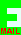## PRIZE PUZZLE FOR DECEMBER 2005

### The Hill Climb

A car climbs a hill at an average speed of 20 miles per hour. What speed must it do going down the hill on the other side so that the overall average speed is 30 miles per hour? Both sides of the hill are the same distance.

Send your answer to me, David Broughton. My email address can be obtained by clicking this symbol.
Closing date: 4th Jan 2006.

(Scroll down to see the answer and who got it right.)

The answer is 60 miles per hour. Did you answer 40? It is true that the average of 40 and 20 is 30 but these are miles per hour over equal distances, not equal time. 40 would be the correct answer if the time up and the time down were the same but the problem makes it clear that the distances up and down are the same and a different approach to the problem is needed. One way is to assume a simple distance, like 60 miles. The answer does not depend on the distance -- you can choose any figure that is convenient.

Half the distance to climb the hill at 20 mph is then 30/20 = 1.5 hours. The average is to be 30 mph so the total time over the total distance of 60 miles is 60/30 = 2 hours. So the down journey must take half an hour (2 minus 1.5). To do 30 miles in half an hour means a speed of 60 mile per hour.

I received eight answers, 7 of them correct. The correct answers were from John Rackett, John Underwood (winner of the draw), Colin Rowe, David Groom, John Stafford, Peter Wreford, and John Bownas. Many thanks for all your answers.

The January Puzzle is another car journey but quite different.

[home] [back]